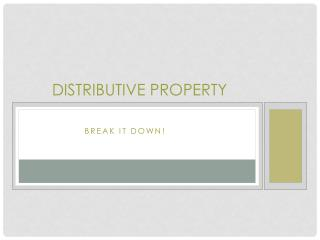DownloadDownload PresentationDistributive Property

# Distributive Property

Télécharger la présentation## Distributive Property

- - - - - - - - - - - - - - - - - - - - - - - - - - - E N D - - - - - - - - - - - - - - - - - - - - - - - - - - -
##### Presentation Transcript

1. Distributive Property Break It Down!

2. Definition of distributive property *The rewritten products are factors of the original numbers. *The original product is equal to the sum/difference of the new products. A product can be rewritten as the sum of two products or the difference between two products. Distributive Property Students often only multiply the first number in the parentheses. 6(4 + 5) ≠ 6(4) + 5

3. Uses for the Distributive Property Works Multiplication 8 x 7 = ? 7 = 5 + 2 So… 8 x (5 + 2) + 40 + 16 56 Step by Step Break down one of the numbers into a sum of two smaller numbers. Then multiply each of the smaller numbers by the multiplier. *Keep the operation the same. 8(5) 8(2)

4. Uses for the Distributive Property Works Multiplication 8 x 9 = ? 9 = 10 - 1 So… 8 x (10 - 1) 80 – 8 72 Step by Step Break down one of the numbers into a difference of two smaller numbers. Then multiply each of the smaller numbers by the multiplier. *Keep the operation the same. 8(10) - 8(1)

5. Uses for the Distributive Property Works Addition of two numbers 72 + 24 = ? 2 is a common factor of 72 & 24. 72 ÷ 2 = 36 24 ÷ 2 = 12 2 x (36 + 12) Step by Step Factor out a common factor from the two numbers. You will need the common factor and the dividends. Rewrite the initial sum as a product of two numbers using the common factor and the dividends. The common factor goes outside the parentheses. The quotients go inside the parentheses

6. Gold digger &the Distributive Property You can use the Gold Digger for the distributive property! Factor the expression 24 + 36 * Factor as if you want to find the Greatest Common Factor. ÷ 24 36 12 18 2 6 9 2 3 3 2

7. Gold digger &the Distributive Property 24 + 36 • Multiply down for the common factor you want to use. • Then use the quotients next to the factor to rewrite the problem. 2(12 + 18) 4(6 + 9) 12(2 + 3) ÷ 24 36 12 18 2 x 4 6 9 2 x 12 3 3 3 2

8. Check your work 24 + 36 24 + 36 = 60 2 X (12 + 18) 24 + 36 = 60 2 x (12 + 18) 24 + 36 = 60 2 x (12 + 18) 24 + 36 = 60

9. Gold digger &the Distributive Property You can use the Gold Digger for the distributive property! Factor the expression 12 + 6 * Factor as if you want to find the Greatest Common Factor. ÷ 12 6 6 3 2 2 1 3

10. Gold digger &the Distributive Property 12 + 6 • Multiply down for the common factor you want to use. • Then use the quotients next to the factor to rewrite the problem. 2(6 + 3) 6(2 + 1) ÷ 12 6 6 3 2 x 6 2 1 3

11. Check your work 12 + 6 12 + 6 = 18 2 X (6 + 3) 12 + 6 = 18 6 x (2 + 1) 12 + 6 = 18

12. Gold digger &the Distributive Property You can use the Gold Digger for the distributive property! Factor the expression 24 + 60 * Factor as if you want to find the Greatest Common Factor. ÷ 24 60 12 30 2 6 15 2 3 5 2

13. Gold digger &the Distributive Property 24 + 60 • Multiply down for the common factor you want to use. • Then use the quotients next to the factor to rewrite the problem. 2(12 + 30) 4(6 + 15) 12(2 + 5) ÷ 24 60 12 30 2 x 4 6 15 2 x 12 3 3 5 2

14. Check your work 24 + 60 24 + 60 = 84 2 X (12 + 30) 24 + 60 = 84 4 x (6 + 10) 24 + 60 = 84 12 x (12 + 30) 24 + 60 = 84

15. Now, You Try! Factor the expression 30 + 45 ÷ 30 45 10 15 3 15 (2 + 3) x 15 2 3 5